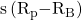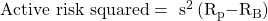Essential Concept 93: Factor Models in Risk Attribution | IFT World
101 Concepts for the Level I Exam

# Essential Concept 93: Factor Models in Risk AttributionActive risk, also known as tracking error or tracking risk, is the standard deviation of active returns. Tracking error is expressed as:whereis the sample standard deviation of the time series of differences between the portfolio return and the benchmark return.

The information ratio is used to measure active returns per unit of active risk. The formula for IR is given by:For example, consider a portfolio that has a sample mean return of 9% and the benchmark has a sample mean return of 7.5%. If the portfolio’s tracking error is 6%, then what is its IR?

IR = (9-7.5)/6 = 0.25

If we use variances rather than standard deviation to measure risk, then the risk measure is called active risk squared. The formula for active risk squared is given as:Active risk squared = Active factor risk + Active specific risk

Active factor risk is the risk due to portfolio’s different-than-benchmark exposures relative to factors specified in the risk model.

Active specific risk are risks resulting from the portfolio’s active weights on individual assets. It is also known as asset selection risk. This is the active non-factor or residual risk.